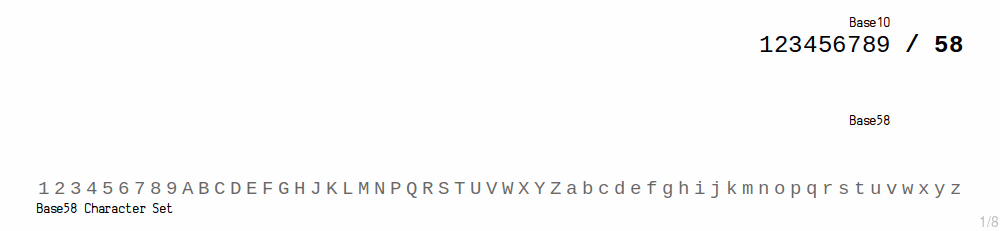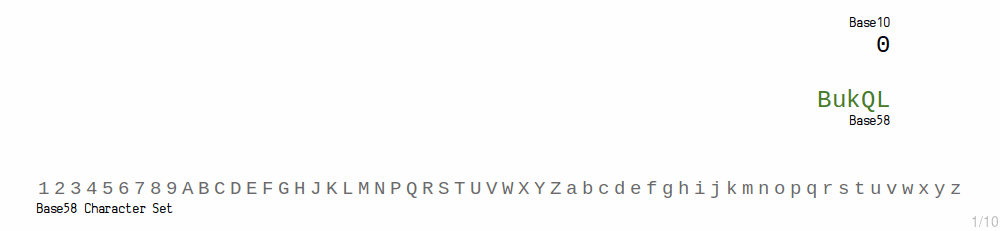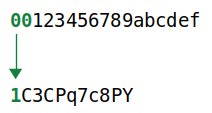• Hash256
• Hash160
• Reverse Bytes
• Satoshis

# Base58

Representing values with an easy-to-share set of characters.

``````  1 2 3 4 5 6 7 8 9
A B C D E F G H   J K L M N   P Q R S T U V W X Y Z
a b c d e f g h i j k   m n o p q r s t u v w x y z``````

Base58 is a set of characters you can use to represent big numbers in a shorter and more user-friendly format.

0d
0x

## What does base58 mean?

The “base” refers to the number of characters you use to represent a value.

Base Characters
2 (binary) `01`
10 (decimal) `0123456789`
16 (hexadecimal) `0123456789abcdef`
58 ` 123456789ABCDEFGH JKLMN PQRSTUVWXYZabcdefghijk mnopqrstuvwxyz`

So in everyday life, we are used to working with base10 numbers (using the digits `0-9`).

But if you’re a computer, it’s easy enough to use extra characters to represent values:

``````base10(9999) = 9999
base16(9999) = 270f
base58(9999) = 3yQ``````

All of these “numbers” have the same valuethey just use different sets of characters (bases) to represent it.

The more characters you have in your base, the less of them you will need to use to represent big numbers. So the bigger your base, the shorter your “number” can be.

## Why base58?

Because 58 is the number of characters you are left with when you use all the characters in the alphanumeric alphabet (62), but remove all the easily mistakable characters like `0`, `O`, `l` and `I`.

``````alphanumeric = 0123456789ABCDEFGHIJKLMNOPQRSTUVWXYZabcdefghijklmnopqrstuvwxyz
base58       =  123456789ABCDEFGH JKLMN PQRSTUVWXYZabcdefghijk mnopqrstuvwxyz``````

1. It gives you a large set of characters, so you can represent large numbers in a shorter format.
2. It leaves out awkward characters, to save you from making mistakes when transcribing.

## Base58 Encode

To convert an integer (base10) to base58, you use the modulus1 function.

Basically, you keep dividing your number by 58, taking the remainder at each step of the way to get the next character index for base58, and finishing when there are no remainders left.

``````base10 = 123456789

123456789 % 58 = 19
2128565 % 58 = 23
36699 % 58 = 43
632 % 58 = 52
10 % 58 = 10

base58 = 
base58 = BukQL``````Base58 Encode (simple)
``````# A simple function that converts an _integer_ to base58:

def int_to_base58(i)

@characters = %w[
1 2 3 4 5 6 7 8 9
A B C D E F G H   J K L M N   P Q R S T U V W X Y Z
a b c d e f g h i j k   m n o p q r s t u v w x y z
]

# create an empty string (in preparation to hold the new characters)
buffer = ''

# keep finding the remainder until our starting number hits zero
while i > 0
# find the remainder after dividing by 58 (% = modulus)
remainder = i % 58

# add the base58 character to the start of the string
buffer = @characters[remainder] + buffer

# divide our integer by 58, and repeat...
i = i / 58
end

return buffer

end

puts int_to_base58(123456789) #=> BukQL``````

## Base58 Decode

To convert a base58 value in to base10, you take each character index and multiply it with how many 58s that position in the number represents.

Then you just add all these values together.

``````base58 = BukQL

L = 19 * 58^0 = 19
Q = 23 * 58^1 = 1334
k = 43 * 58^2 = 144652
u = 52 * 58^3 = 10145824
B = 10 * 58^4 = 113164960

base10 = 19 + 1334 + 144652 + 10145824 + 113164960
base10 = 123456789``````Base58 Decode (simple)
``````def base58_to_int(base58)

@characters = %w[
1 2 3 4 5 6 7 8 9
A B C D E F G H   J K L M N   P Q R S T U V W X Y Z
a b c d e f g h i j k   m n o p q r s t u v w x y z
]

# create an integer to hold the result
total = 0

# reverse the base58 string so we can read characters from right to left
base58 = base58.reverse

# run through each character, including the index so we know how many character we've read
base58.each_char.with_index do |char, i|

# get the index number for this character
char_i = @characters.index(char)

# work out how many 58s this character represents (increment the power for each character)
value  = char_i * (58**i)

total = total + value
end

end

puts base58_to_int("BukQL") #=> 123456789``````

## Base58 in Bitcoin

Base58 is used in bitcoin when you want to convert commonly used data in to an easier-to-share format. For example:

1. WIF Private Keys
• A private key is like a “master password”, and you can use it when you want to import bitcoins in to a new wallet. For this occasion, there is such a thing as a WIF Private Key, which is basically a private key in base58.
• A public key is the “public” counterpart to a private key, and you use them when you want to send bitcoins to someone, so it’s expected that you’re going to type one out from time to time. However, public keys are quite lengthy, so we convert them to Addresses instead, which makes use of base58 in the final step of the conversion.

### Leading Zeros (`0x00…`)

0x: A `0x` prefix indicates a hexadecimal value. Hexadecimal values will sometimes only contain the numbers 0-9 and could therefore be confused as being decimal values, so the prefix helps us to distinguish between them. This prefix is discarded before being used in calculation.

Byte: A byte of data can hold a value of between `0-255`, and can be represented by two hexadecimal characters. For example, `0xff` is one byte of data and represents the value `255` in decimal.

We convert every zero byte (`0x00`) at the start of a hexadecimal value to a `1` in base58.You see, putting zeros at the start of a number does not increase the size of it (e.g. `0x12` is the same as `0x0012`), so when we convert to base58 (which uses the modulus function) any extra zeros at the start will not affect the result.

Therefore, to ensure that leading zeros have an influence on the result, the bitcoin base58 encoding includes a manual step to convert all leading `0x00`’s to `1`’s.

I’m not sure why we convert zero bytes at the start to `1`s in base58, but that’s how it works in bitcoin.

### Prefixes

In Bitcoin, different prefixes are added to data before converting to base58 to influence the leading character of the result. This leading character then helps us to identify what each base58 string represents.

These are the most common prefixes used in bitcoin:

Mainnet
Prefix (hex) Base58 Leading Character Represents Example
`00` 1 P2PKH Address `1AKDDsfTh8uY4X3ppy1m7jw1fVMBSMkzjP`
`05` 3 P2SH Address `34nSkinWC9rDDJiUY438qQN1JHmGqBHGW7`
`80` K / L WIF Private Key`L4mee2GrpBSckB9SgC9WhHxvtEgKUvgvTiyYcGu38mr9CGKBGp93`
`80` 5 WIF Private Key`5KXWNXeaVMwjzMsrKPv8dmdEZuVPmPay4nm5SfVZCjLHoy1B56w`
`0488ADE4` xprv Extended Private Key `xprv9tuogRdb5YTgcL3P8Waj7REqDuQx4sXcodQaWTtEVFEp6yRKh1CjrWfXChnhgHeLDuXxo2auDZegMiVMGGxwxcrb2PmiGyCngLxvLeGsZRq`
`0488B21E` xpub Extended Public Key `xpub67uA5wAUuv1ypp7rEY7jUZBZmwFSULFUArLBJrHr3amnymkUEYWzQJz13zLacZv33sSuxKVmerpZeFExapBNt8HpAqtTtWqDQRAgyqSKUHu`
Testnet
Prefix (hex) Base58 Leading Character Represents Example
`6F` m / n P2PKH Address `ms2qxPw1Q2nTkm4eMHqe6mM7JAFqAwDhpB`
`C4` 2 P2SH Address `2MwSNRexxm3uhAKF696xq3ztdiqgMj36rJo`
`EF` c WIF Private Key`cV8e6wGiFF8succi4bxe4cTzWTyj9NncXm81ihMYdtW9T1QXV5gS`
`EF` 9 WIF Private Key`93J8xGU85b1sxRP8wjp3WNBCDZr6vZ8AQjd2XHr4YU5Lb21jS1L`
`04358394` tprv Extended Private Key `tprv9tuogRdb5YTgcL3P8Waj7REqDuQx4sXcodQaWTtEVFEp6yRKh1CjrWfXChnhgHeLDuXxo2auDZegMiVMGGxwxcrb2PmiGyCngLxvLeGsZRq`
`043587CF` tpub Extended Public Key `tpub67uA5wAUuv1ypp7rEY7jUZBZmwFSULFUArLBJrHr3amnymkUEYWzQJz13zLacZv33sSuxKVmerpZeFExapBNt8HpAqtTtWqDQRAgyqSKUHu`

As mentioned the hex prefix `00` does not naturally get converted to a “1” when encoding to base58. This conversion is performed manually in the code instead.

You’ll notice that WIF Private Keys use the same hex prefix, but produce different leading characters. This is because if a private key is used to create a compressed public key (which will produce a different address to an uncompressed public key), we also append a `01` to it before converting to base58. This extra byte has an effect on the leading character in the base58 result.

Extended Keys contain extra metadata alongside the original public and private keys, which is why their base58 strings are much longer.

## Code

These code snippets do the base58 conversion used in Bitcoin. They convert to and from hexadecimal, because that’s the most common format we work with.

### Ruby

``````module Base58

@chars = %w[
1 2 3 4 5 6 7 8 9
A B C D E F G H   J K L M N   P Q R S T U V W X Y Z
a b c d e f g h i j k   m n o p q r s t u v w x y z
]
@base = @chars.length

def self.encode(hex)
i = hex.to_i(16)
buffer = String.new

while i > 0
remainder = i % @base
i = i / @base
buffer = @chars[remainder] + buffer
end

# add '1's to the start based on number of leading bytes of zeros
leading_zero_bytes = (hex.match(/^(+)/) ? \$1 : '').size / 2

end

def self.decode(base58)
total = 0 # integer to hold conversion to decimal

# run through each character
base58.reverse.each_char.with_index do |char, i|
char_i = @chars.index(char) # get the index number for this character
value  = (58**i) * char_i   # work out how many 58s this character represents
total = total + value     # add to total
end

# convert this integer to hex
hex = total.to_s(16)

leading_1s = (base58.match(/^(+)/) ? \$1 : '').size

end

end

puts Base58.encode('0093ce48570b55c42c2af816aeaba06cfee1224faebb6127fe') #=> 1EUXSxuUVy2PC5enGXR1a3yxbEjNWMHuem
puts Base58.decode('1EUXSxuUVy2PC5enGXR1a3yxbEjNWMHuem') #=> 0093ce48570b55c42c2af816aeaba06cfee1224faebb6127fe``````

### PHP

``````<?php

// Sample Input
\$hex = "00662ad25db00e7bb38bc04831ae48b4b446d1269817d515b6"; // a public key hash (with a 00 prefix)

// -------------
// Base58 Encode
// -------------
// Convert hex string to an integer
\$num = gmp_init(\$hex, 16);
\$base58 = "";

// Base58 Characters
\$chars = str_split("123456789ABCDEFGHJKLMNPQRSTUVWXYZabcdefghijkmnopqrstuvwxyz");

// Keep dividing by 58 and taking the remainder as the character
while (\$num > 0) {
\$rem = gmp_mod(\$num, 58); // remainder (what we get the character for)
\$num = gmp_div(\$num, 58); // quotient  (keep dividing the number to get remainders)
\$base58 = \$chars[intval(\$rem)].\$base58; // add base58 char to the start
}

// Convert leading 00s in hex to leading 1s (this is done manually in the base58 conversion)
\$count = intval(strspn(\$hex, "0") / 2); // how many leading 0s, then divide by 2 (to work out how many zero bytes have been prefixed)
\$leading = str_repeat("1", \$count); // prefix one leading 1 for every zero byte (e.g. 00)

// Result
echo \$result.PHP_EOL; // 1AKDDsfTh8uY4X3ppy1m7jw1fVMBSMkzjP

// -------------
// Base58 Decode
// -------------
\$base58 = "1AKDDsfTh8uY4X3ppy1m7jw1fVMBSMkzjP";
\$int = gmp_init(0); // integer to hold result

// Convert to decimal
\$base58a = str_split(strrev(\$base58));   // create an array we can loop through
foreach (\$base58a as \$i => \$c) {         // run through each character
\$multiple = gmp_pow(58, \$i);         // how many 58s this position holds (e.g. 58^0, 58^1, 58^2...)
\$index = array_search(\$c, \$chars);   // get index number for base58 char (e.g. B=10)
\$value = gmp_mul(\$index, \$multiple); // multiply to get number of 58s this character is representing
\$int = \$int + \$value;                // add to total
}

\$gmp = gmp_init(strval(\$int), 10); // create gmp number from bit string (base 10) NOTE: gmp_init takes strings
\$hex = gmp_strval(\$gmp, 16); // convert to hex string representation
if (strlen(\$hex) % 2 !== 0) { // return even number of characters (hex2bin prefers it)
\$hex = '0'.\$hex;
}

// Convert leading 1s in base58 to leading 00s (this is done manually in the base58 conversion)
\$count = strspn(\$base58, "1");

// Result

### Modulus (%)

The modulus (`%`) function is a sister of the divide (`/`) function. It gives you the remainder of a division:

``````7 % 6 = 1
7 % 5 = 2
7 % 4 = 3
7 % 3 = 1``````

### Source

• `src/base58.cpp`